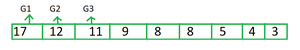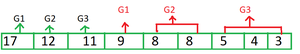# Maximize sum of minimum and maximum of all groups in distribution

• Last Updated : 28 Jan, 2022

Given an array arr[], and an integer N. The task is to maximize the sum of minimum and maximum of each group in a distribution of the array elements in N groups where the size of each group is given in an array b[] of size N.

Examples:

Input: a[] = {17, 12, 11, 9, 8, 8, 5, 4, 3}, N = 3,
b[] = {2, 3, 4}
Output: 60
Explanation: The array elements should be distributed in groups {17, 9} {12, 8, 8} {11, 5, 4, 3}.
So the sum becomes (17 + 9) + (12 + 8) + (11 + 3) = 26 + 20 + 14 = 60

Input: a[] = {12, 3, 4, 2, 5, 9, 8, 1, 2}, N = 3,
b[] = {1, 4, 4}
Output: 45

Approach: This problem can be solved by using the Greedy Approach and some implementation. Follow the steps below to solve the given problem.

• sort array arr[] in descending order and b[] in ascending order.
• Initialize a variable ans to store the output.
• Iterate from i = 0 to i = N-1 in array a[] and add each element to ans.
• Initialize a variable ind to store the index of elements of the array arr[]. Assign N to variable ind.
• Take a loop from i=0 to N-1.
• If b[i] > 0 then increment ind with (b[i]-1)
• Add arr[ind] to ans, as arr[ind] will be the smallest element of that group
• increment ind with 1.
• output the ans.

See the illustration below for better understanding.

Illustration:

Consider an example: arr[] = {17, 12, 11, 9, 8, 8, 5, 4, 3}, N = 3 and b[] = {2, 3, 4}

Firstly, sort array arr[] in descending order and b[] in ascending order, After then put the first N greatest element of array arr[] to each group as shown in fig.Secondly, Fill each group with the rest of the element of array arr[] (one group at a time)Therefore answer will contain the sum of the first N elements of array arr[]  i.e. 17, 12, 11 and also the last element which is filled in each group i.e. 9, 8 and 3.

Below is the implementation of the above approach.

## C++

 `// C++ program for above approach` `#include ` `using` `namespace` `std;`   `// Function to maximum possible sum of minimum` `// and maximum elements of each group` `int` `geeksforgeeks(``int` `a[], ``int` `b[], ``int` `n, ``int` `k)` `{` `    ``// Sorting array a in descending order` `    ``// and array a in ascending order.` `    ``sort(a, a + n, greater<``int``>());`   `    ``sort(b, b + k);`   `    ``// Variable to store the required output.` `    ``int` `ans = 0;`   `    ``// Loop to store sum of first k greatest` `    ``// element of array a[] in ans.` `    ``// since they will be gretest element` `    ``// of each group when distributed` `    ``// in group one by one.` `    ``for` `(``int` `i = 0; i < k; i++) {` `        ``if` `(b[i] == 1) {` `            ``ans += 2 * a[i];` `        ``}` `        ``else` `{` `            ``ans += a[i];` `        ``}`   `        ``--b[i];` `    ``}` `    ``// Variable to store index of element a .` `    ``int` `ind = k;`   `    ``// Then after when each grouped is filled,` `    ``// then add a[ind] to ans as a[ind] will be` `    ``// lowest element of each group.` `    ``for` `(``int` `i = 0; i < k; i++) {` `        ``if` `(b[i] > 0) {` `            ``ind += b[i] - 1;` `            ``ans += a[ind];` `            ``ind++;` `        ``}` `    ``}`   `    ``return` `ans;` `}`   `// Driver code` `int` `main()` `{`   `    ``int` `N = 3;`   `    ``// Size of array a[]` `    ``int` `siz = 9;`   `    ``int` `a[] = { 17, 12, 11, 9, 8, 8, 5, 4, 3 };` `    ``int` `b[] = { 2, 3, 4 };`   `    ``// Function Call` `    ``cout << geeksforgeeks(a, b, 9, N);` `}`

## Java

 `// Java code to find the maximum median` `// of a sub array having length at least K.` `import` `java.util.*;` `public` `class` `GFG` `{`   `// Utility function to sort an array in` `// descending order` `static` `void` `sort(``int` `arr[])` `{` `    ``for` `(``int` `i = ``0``; i < arr.length; i++)` `    ``{     ` `        ``for` `(``int` `j = i + ``1``; j < arr.length; j++)` `        ``{     ` `              ``if``(arr[i] < arr[j])` `              ``{    ` `                  ``int` `temp = arr[i];    ` `                  ``arr[i] = arr[j];    ` `                  ``arr[j] = temp;    ` `              ``}     ` `        ``}     ` `    ``}    ` `}`   `// Function to maximum possible sum of minimum` `// and maximum elements of each group` `static` `int` `geeksforgeeks(``int` `a[], ``int` `b[], ``int` `n, ``int` `k)` `{` `    ``// Sorting array a in descending order` `    ``// and array b in ascending order.` `    ``sort(a);`   `    ``Arrays.sort(b);`   `    ``// Variable to store the required output.` `    ``int` `ans = ``0``;`   `    ``// Loop to store sum of first k greatest` `    ``// element of array a[] in ans.` `    ``// since they will be gretest element` `    ``// of each group when distributed` `    ``// in group one by one.` `    ``for` `(``int` `i = ``0``; i < k; i++) {` `        ``if` `(b[i] == ``1``) {` `            ``ans += ``2` `* a[i];` `        ``}` `        ``else` `{` `            ``ans += a[i];` `        ``}`   `        ``--b[i];` `    ``}` `    ``// Variable to store index of element a .` `    ``int` `ind = k;`   `    ``// Then after when each grouped is filled,` `    ``// then add a[ind] to ans as a[ind] will be` `    ``// lowest element of each group.` `    ``for` `(``int` `i = ``0``; i < k; i++) {` `        ``if` `(b[i] > ``0``) {` `            ``ind += b[i] - ``1``;` `            ``ans += a[ind];` `            ``ind++;` `        ``}` `    ``}`   `    ``return` `ans;` `}`   `// Driver code` `public` `static` `void` `main(String args[])` `{` `    ``int` `N = ``3``;`   `    ``// Size of array a[]` `    ``int` `siz = ``9``;`   `    ``int` `a[] = { ``17``, ``12``, ``11``, ``9``, ``8``, ``8``, ``5``, ``4``, ``3` `};` `    ``int` `b[] = { ``2``, ``3``, ``4` `};`   `    ``// Function Call` `    ``System.out.println(geeksforgeeks(a, b, ``9``, N));` `}` `}`   `// This code is contributed by Samim Hossain Mondal.`

## Python

 `# Python program for the above approach`   `# Function to maximum possible sum of minimum` `# and maximum elements of each group` `def` `geeksforgeeks(a, b, n, k):` `    `  `    ``# Sorting array a in descending order` `    ``# and array a in ascending order.` `    ``a.sort(reverse``=``True``)`   `    ``b.sort()`   `    ``# Variable to store the required output.` `    ``ans ``=` `0`   `    ``# Loop to store sum of first k greatest` `    ``# element of array a[] in ans.` `    ``# since they will be gretest element` `    ``# of each group when distributed` `    ``# in group one by one.` `    ``for` `i ``in` `range``(``0``, k):` `        ``if` `(b[i] ``=``=` `1``):` `            ``ans ``=` `ans ``+` `(``2` `*` `a[i])` `        `  `        ``else``:` `            ``ans ``=` `ans ``+` `a[i]`   `        ``b[i] ``=` `b[i] ``-` `1` `        `  `    ``# Variable to store index of element a .` `    ``ind ``=` `k`   `    ``# Then after when each grouped is filled,` `    ``# then add a[ind] to ans as a[ind] will be` `    ``# lowest element of each group.` `    ``for` `i ``in` `range``(``0``, k):` `        ``if` `(b[i] > ``0``):` `            ``ind ``=` `ind ``+` `b[i] ``-` `1` `            ``ans ``=` `ans ``+` `a[ind]` `            ``ind ``=` `ind ``+` `1`   `    ``return` `ans`   `# Driver code` `N ``=` `3`   `# Size of array a[]` `siz ``=` `9``;`   `a ``=` `[ ``17``, ``12``, ``11``, ``9``, ``8``, ``8``, ``5``, ``4``, ``3` `]` `b ``=` `[ ``2``, ``3``, ``4` `]`   `# Function Call` `print``(geeksforgeeks(a, b, ``9``, N))`   `# This code is contributed by Samim Hossain Mondal.`

## C#

 `// C# code to find the maximum median` `// of a sub array having length at least K.` `using` `System;` `public` `class` `GFG` `{`   `  ``// Utility function to sort an array in` `  ``// descending order` `  ``static` `void` `sort(``int``[] arr)` `  ``{` `    ``for` `(``int` `i = 0; i < arr.Length; i++)` `    ``{     ` `      ``for` `(``int` `j = i + 1; j < arr.Length; j++)` `      ``{     ` `        ``if``(arr[i] < arr[j])` `        ``{    ` `          ``int` `temp = arr[i];    ` `          ``arr[i] = arr[j];    ` `          ``arr[j] = temp;    ` `        ``}     ` `      ``}     ` `    ``}    ` `  ``}`   `  ``// Function to maximum possible sum of minimum` `  ``// and maximum elements of each group` `  ``static` `int` `geeksforgeeks(``int``[] a, ``int``[] b, ``int` `n, ``int` `k)` `  ``{` `    `  `    ``// Sorting array a in descending order` `    ``// and array b in ascending order.` `    ``sort(a);`   `    ``Array.Sort(b);`   `    ``// Variable to store the required output.` `    ``int` `ans = 0;`   `    ``// Loop to store sum of first k greatest` `    ``// element of array a[] in ans.` `    ``// since they will be gretest element` `    ``// of each group when distributed` `    ``// in group one by one.` `    ``for` `(``int` `i = 0; i < k; i++) {` `      ``if` `(b[i] == 1) {` `        ``ans += 2 * a[i];` `      ``}` `      ``else` `{` `        ``ans += a[i];` `      ``}`   `      ``--b[i];` `    ``}` `    ``// Variable to store index of element a .` `    ``int` `ind = k;`   `    ``// Then after when each grouped is filled,` `    ``// then add a[ind] to ans as a[ind] will be` `    ``// lowest element of each group.` `    ``for` `(``int` `i = 0; i < k; i++) {` `      ``if` `(b[i] > 0) {` `        ``ind += b[i] - 1;` `        ``ans += a[ind];` `        ``ind++;` `      ``}` `    ``}`   `    ``return` `ans;` `  ``}`   `  ``// Driver code` `  ``public` `static` `void` `Main()` `  ``{` `    ``int` `N = 3;`   `    ``// Size of array a[]` `    ``int` `siz = 9;`   `    ``int``[] a = { 17, 12, 11, 9, 8, 8, 5, 4, 3 };` `    ``int``[] b = { 2, 3, 4 };`   `    ``// Function Call` `    ``Console.Write(geeksforgeeks(a, b, 9, N));` `  ``}` `}`   `// This code is contributed by Saurabh Jaiswal`

## Javascript

 ``

Output

`60`

Time complexity: O(M * logM) where M is the size of array arr.
Auxiliary Space: O(1)

My Personal Notes arrow_drop_up
Recommended Articles
Page :